## How Many Pairs of Rabbits Are Created by One Pair in One Year?

The Fibonacci numbers are often illustrated geometrically, with spirals or square tilings, but the nautilus is not their origin. I recently learned that the sequence was first reported as the solution to a dynamic modeling thought experiment, posed by Leonardo Pisano (Fibonacci) in his 1202 masterpiece, Liber Abaci.

How Many Pairs of Rabbits Are Created by One Pair in One Year?

A certain man had one pair of rabbits together in a certain enclosed place, and one wishes to know how many are created from the pair in one year when it is the nature of them in a single month to bear another pair, and in the second month those born to bear also. Because the abovewritten pair in the first month bore, you will double it; there will be two pairs in one month. One of these, namely the first, bears in the second month, and thus there are in the second month 3 pairs; of these in one month two are pregnant, and in the third month 2 pairs of rabbits are born, and thus there are 5 pairs in the month; in this month 3 pairs are pregnant, and in the fourth month there are 8 pairs, of which 5 pairs bear another 5 pairs; these are added to the 8 pairs making 13 pairs in the fifth month; these 5 pairs that are born in this month do not mate in this month, but another 8 pairs are pregnant, and thus there are in the sixth month 21 pairs; [p284] to these are added the 13 pairs that are born in the seventh month; there will be 34 pairs in this month; to this are added the 21 pairs that are born in the eighth month; there will be 55 pairs in this month; to these are added the 34 pairs that are born in the ninth month; there will be 89 pairs in this month; to these are added again the 55 pairs that are born in the tenth month; there will be 144 pairs in this month; to these are added again the 89 pairs that are born in the eleventh month; there will be 233 pairs in this month.

Source: http://www.math.utah.edu/~beebe/software/java/fibonacci/liber-abaci.html

The solution is the famous Fibonacci sequence, which can be written as a recurrent series,

`F(n) = F(n-1)+F(n-2), F(0)=F(1)=1`

This can be directly implemented as a discrete time Vensim model: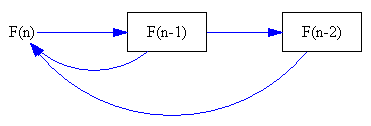However, that representation is a little too abstract to immediately reveal the connection to rabbits. Instead, I prefer to revert to Fibonacci’s problem description to construct an operational representation: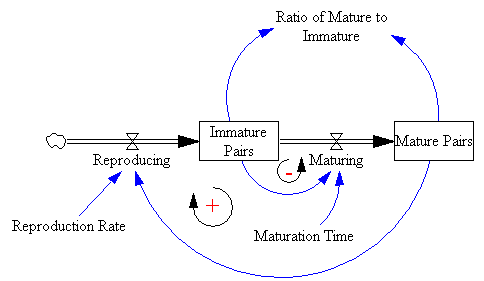Mature rabbit pairs are held in a stock (Fibonacci’s “certain enclosed space”), and they breed a new pair each month (i.e. the Reproduction Rate = 1/month). Modeling male-female pairs rather than individual rabbits neatly sidesteps concern over the gender mix. Importantly, there’s a one-month delay between birth and breeding (“in the second month those born to bear also”). That delay is captured by the Immature Pairs stock. Rabbits live forever in this thought experiment, so there’s no outflow from mature pairs.

You can see the relationship between the series and the stock-flow structure if you write down the discrete time representation of the model, ignoring units and assuming that the TIME STEP = Reproduction Rate = Maturation Time = 1:

`Mature Pairs(t) = Mature Pairs(t-1) + Maturing`
`Immature Pairs(t) = Immature Pairs(t-1) + Reproducing - Maturing`

Substituting Maturing = Immature Pairs and Reproducing = Mature Pairs,

`Mature Pairs(t) = Mature Pairs(t-1) + Immature Pairs(t-1)`
`Immature Pairs(t) = Immature Pairs(t-1) + Mature Pairs(t-1) - Immature Pairs(t-1) = Mature Pairs(t-1)`

So:

`Mature Pairs(t) = Mature Pairs(t-1) + Mature Pairs(t-2)`

The resulting model has two feedback loops: a minor negative loop governing the Maturing of Immature Pairs, and a positive loop of rabbits Reproducing. The rabbit population tends to explode, due to the positive loop: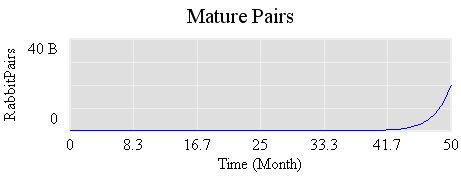In four years, there are about as many rabbits as there are humans on earth, so that “certain enclosed space” better be big. After an initial transient, the growth rate quickly settles down: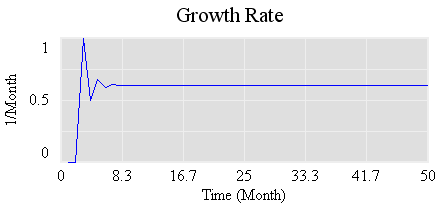Its steady-state value is .61803… (61.8%/month), which is the Golden Ratio conjugate. If you change the variable names, you can see the relationship to the tiling interpretation and the Golden Ratio: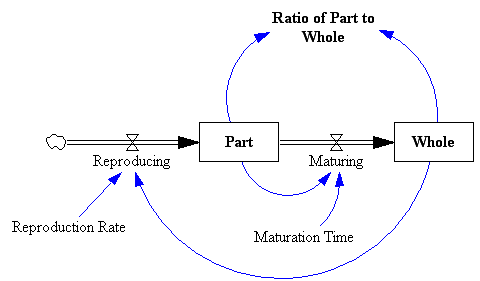Like anything that grows exponentially, the Fibonacci numbers get big fast. The hundredth is  354,224,848,179,261,915,075.

As before, we can play the eigenvector trick to suppress the growth mode. The system is described by the matrix:

```-1 1
1 0```

which has eigenvalues {-1.618033988749895, 0.6180339887498949} – notice the appearance of the Golden Ratio. If we initialize the model with the eigenvector of the negative eigenvalue, {-0.8506508083520399, 0.5257311121191336}, we can get the bunny population under control, at least until numerical noise excites the growth mode, near time 25: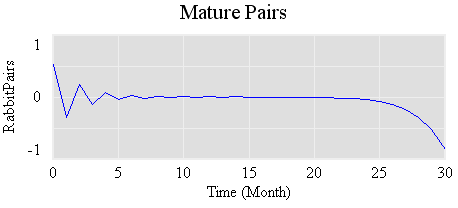The problem is that we need negarabbits to do it, -.850653 immature rabbits initially, so this is not a physically realizable solution (which probably guarantees that it will soon be introduced in legislation).

I brought this up with my kids, and they immediately went to the physics of the problem: “Rabbits don’t live forever. How big is your cage? Do you have rabbit food? TONS of rabbit food? What if you have all males, or varying mixtures of males and females?”

It’s easy to generalize the structure to generate other sequences. For example, assuming that mature rabbits live for only two months yields the Padovan sequence. Its equivalent of the Golden Ratio is 1.3247…, i.e. the rabbit population grows more slowly at ~32%/month, as you’d expect since rabbit lives are shorter.

The model’s in my library.